Home

# The log P - $\langle M_{K}\rangle$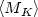Relation of Galactic Field RR Lyrae Variables

## Abstract

The kinematical parameters of the local field RR Lyrae population and the zero-point of the log $P-\langle M_K\rangle$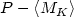relation for these variables are inferred by applying the statistical parallax (maximum-likeli-hood) technique to a sample of 182 RR Lyraes with known periods, radial-velocities, metallicities, K-band photometry, and absolute proper motions on the ICRS system. Hipparcos, Tycho-2, SPM, UCAC, NPM1, and the Four-Million Star Catalog (Volchkov et al. 1992) were used as the sources of proper motions; the proper motions of the last two catalogs are reduced to the Hipparcos (ICRS) system (Dambis & Rastorguev 2001). The K-band magnitudes were adopted from the list of Fernley et al. (1998) and supplemented by the data of the 2MASS Second Incremental Data Release. The parameters of the velocity distribution are found to be: ( $U_0 = -10 \pm 10$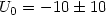, $V_0 = -51 \pm 8$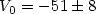, $W_0 = -14 \pm 5)$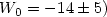km s-1; ( $\sigma_U = 62 \pm 10$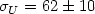, $\sigma_V = 45 \pm 8$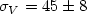, $\sigma_W = 28 \pm 6$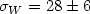) km s-1: and ( $U_0 = -23 \pm 13$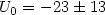, $V_0 = -213 \pm 12$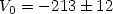, $W_0 = -5 \pm 8$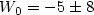) km s-1; ( $\sigma_U = 157 \pm 12$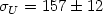, $\sigma_V = 98 \pm 8$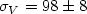, $\sigma_W = 91 \pm 7$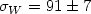) km s-1 for the thick-disk (41 stars) and halo (141 stars) objects, respectively. The zero-point of the infrared PL relation of Jones et al. (1992) (based on the results obtained using the Baade-Wesselink method) is confirmed: we find $\langle M_K\rangle = -2.33 \cdot \log P_{\rm F}-0.82 \pm 0.12$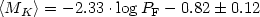compared to $\langle M_K\rangle = -2.33 \cdot \log P_{\rm F}-0.88$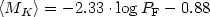as inferred by Jones et al. (1992). A conversion of the resulting log $P-\langle M_K\rangle$relation to V-band luminosities yields the metallicity-luminosity relation $\langle M_V\rangle = +1.04 + 0.14 \cdot [$Fe/H $] \pm 0.11$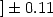. Our results imply a solar Galactocentric distance of $R_0 = 7.6 \pm 0.4$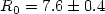kpc and an LMC distance modulus of $DM_{\rm LMC} = 18.18 \pm 0.12$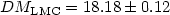(cluster RR Lyraes) or $DM_{\rm LMC} = 18.11 \pm 0.12$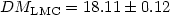(field RR Lyraes), thereby favoring the so-called short distance scale.

# The log P - $\langle M_{K}\rangle$Relation of Galactic Field RR Lyrae Variables

## Metrics

### Full text viewsFull text views reflects the number of PDF downloads, PDFs sent to Google Drive, Dropbox and Kindle and HTML full text views.

Total number of HTML views: 0
Total number of PDF views: 0 *Loading metrics...

### Abstract viewsAbstract views reflect the number of visits to the article landing page.

Total abstract views: 0 *Loading metrics...

* Views captured on Cambridge Core between <date>. This data will be updated every 24 hours.

Usage data cannot currently be displayed